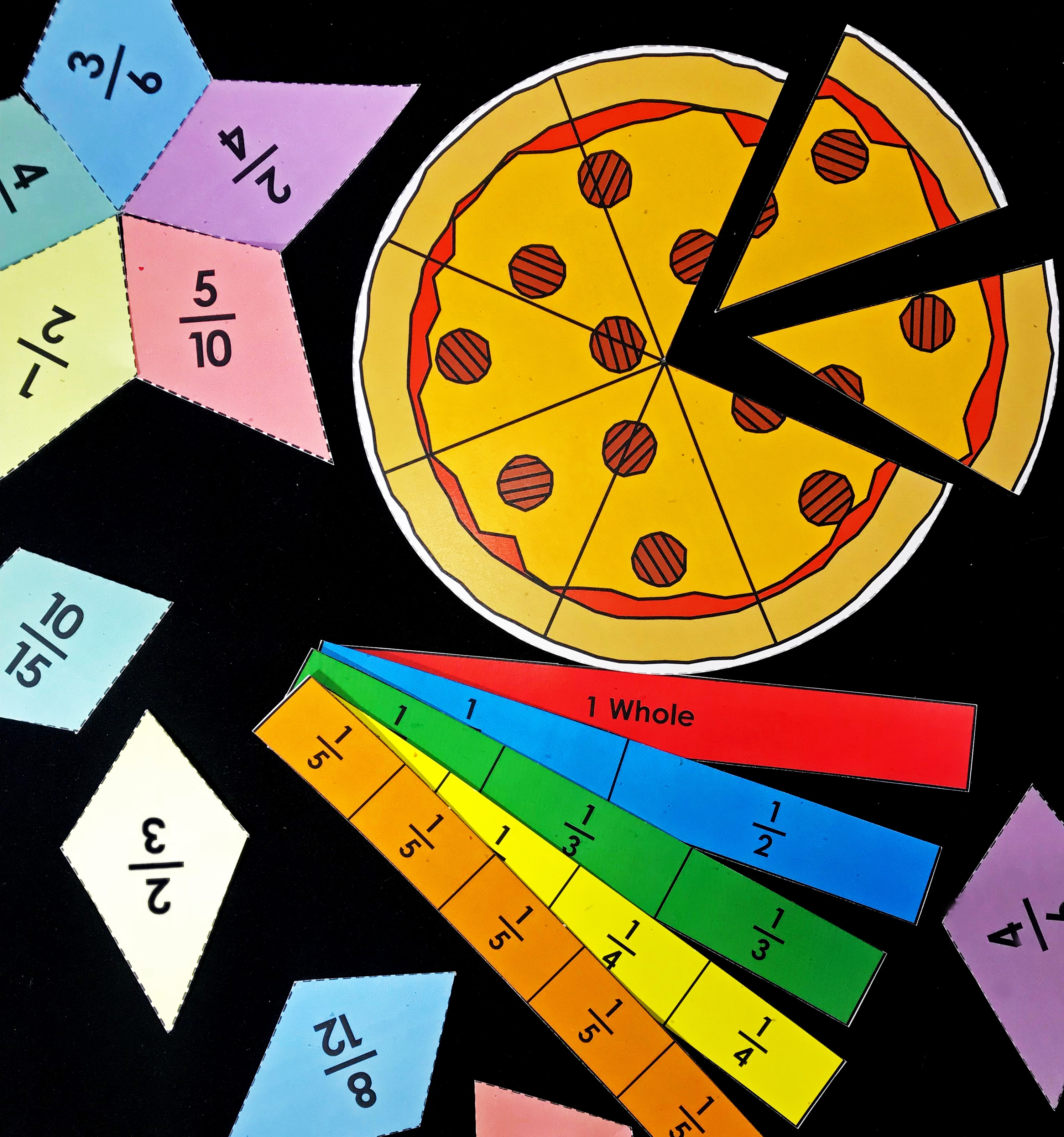# Manipulative Fraction ToolsBrowse the Super Teacher Worksheets fractions collection for useful manipulative fraction tools, including fraction strips, fraction pizzas, fraction circles, and equivalent fraction stars. We have printable resources for a variety of fraction topics, such as basic fractions, equivalent fractions, comparing and ordering fractions, fractions of sets, reciprocal fractions, mixed numbers and improper fractions, and more! Check out our fraction worksheets collection today!